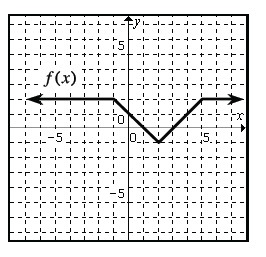### Home > PC > Chapter 1 > Lesson 1.1.4 > Problem1-49

1-49.

Using the graph of $f\left(x\right)$ to the right, state the transformation and make a sketch of the transformed graph. Place each graph on its own set of axes.

1. $f\left(x + 2\right) + 1$

2. $2 f\left(x\right) + 2$

3. $−f\left(x + 4\right)$Use the eTool below to help you with this problem.
Click the link at right for the full version of the eTool: PCT 1-49 HW eTool